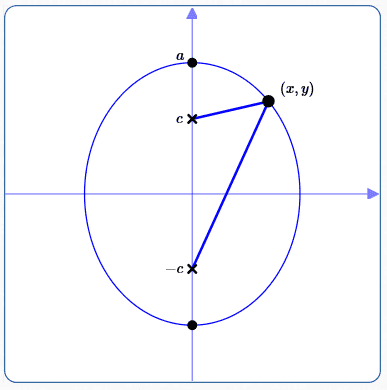﻿ Equations of Ellipses in Standard Form: Foci on the y-axis

# Equations of Ellipses in Standard Form: Foci on the $y$-axis

• PRACTICE (online exercises and printable worksheets)

In an earlier lesson, the equation of an ellipse with center at the origin and foci on the $x$-axis was derived, in great detail.

You may want to review this earlier lesson before studying the ‘in-a-nutshell’ derivation here.

## Derivation of Ellipse Equation: Center at the Origin, Foci on the $y$-axis

Although it is much shorter, this derivation should look strikingly familiar to the earlier derivation.

Notice also that the variables $\,a\,$, $\,b\,$ and $\,c\,$ have the same meaning as in the earlier derivation,
and the relationship between these three variables is the same.

 Position the ellipse: As shown at right, position an ellipse with center at the origin and foci (marked with ‘x’) on the $y$-axis. Notation ($\,c\,$ and $\,a\,$): Define: $c = \,$ the distance from the center (the origin) to each focus. Since $\,c\,$ is a distance, $\,c > 0\,$. The coordinates of the foci are therefore $\,(0,c)\,$ and $\,(0,-c)\,$. $a = \,$ the distance from the center to each vertex. Since $\,a\,$ is a distance, $\,a > 0\,$. The coordinates of the vertices are therefore $\,(0,a)\,$ and $\,(0,-a)\,$. Ellipse constant: The ellipse constant is the length of the major axis, which is $\,2a\,$. Use the definition of ellipse on a typical point: Let $\,(x,y)\,$ be a typical point on the ellipse, so that the sum of its distances to the foci is the ellipse constant ($\,2a\,$). That is, $$\biggl( \text{distance from } (x,y) \text{ to } (0,-c) \biggr) + \biggl( \text{distance from } (x,y) \text{ to } (0,c) \biggr) = 2a$$ Use the distance formula and make obvious simplications: $$\begin{gather} \sqrt{\bigl(x - 0\bigr)^2 + \bigl(y-(-c)\bigr)^2} + \sqrt{\bigl(x - 0\bigr)^2 + \bigl(y-c\bigr)^2} = 2a\cr\cr \sqrt{x^2 + (y+c)^2} + \sqrt{x^2 + (y-c)^2} = 2a \end{gather}$$• Isolate, undo, repeat:
\begin{alignat}{2} &\sqrt{x^2 + (y+c)^2} = 2a - \sqrt{x^2 + (y-c)^2}&\qquad&\text{(isolate the first square root)}\cr\cr &x^2 + (y+c)^2 = 4a^2 - 4a\sqrt{x^2 + (y-c)^2} + x^2 + (y-c)^2&&\text{(square both sides and simplify)}\cr\cr &x^2 + y^2 + 2cy + c^2 = 4a^2 - 4a\sqrt{x^2 + (y-c)^2} + x^2 + y^2 - 2cy + c^2&&\text{(FOIL)}\cr\cr &2cy = 4a^2 - 4a\sqrt{x^2 + (y-c)^2} - 2cy &&\text{(addition property of equality)}\cr\cr &a\sqrt{x^2 + (y-c)^2} = a^2 - cy &&\text{(isolate square root term and divide by \,4)}\cr\cr &a^2 \bigl( x^2 + (y-c)^2 \bigr) = (a^2 - cy)^2 &&\text{(square both sides and simplify)}\cr\cr &a^2 \bigl( x^2 + y^2 - 2cy + c^2 \bigr) = a^4 - 2a^2cy + c^2y^2 &&\text{(FOIL)}\cr\cr &a^2x^2 + a^2y^2 - 2a^2cy + a^2c^2 = a^4 - 2a^2cy + c^2y^2&&\text{(distributive law)}\cr\cr &a^2x^2 + a^2y^2 - c^2y^2 = a^4 - a^2c^2 &&\text{(addition property of equality and re-arrange)}\cr\cr &a^2x^2 + (a^2 - c^2)y^2 = a^2(a^2 - c^2)&&\text{(factor)}\cr\cr \end{alignat}
 Define $\,b\,$; get relationship between $\,a\,$, $\,b\,$ and $\,c\,$: As before, define $\,b\,$ as the distance from the center to an endpoint of the minor axis. Then, $\,c^2 + b^2 = a^2\,$. Equivalently, $\,b^2 = a^2 - c^2\,$ and $\,c^2 = a^2 - b^2\,$. Again, $\,a > b\,$. Rewrite equation to eliminate $\,c\,$, and simplify: $$\begin{gather} a^2x^2 + b^2y^2 = a^2b^2\cr\cr \frac{a^2x^2}{a^2b^2} + \frac{b^2y^2}{a^2b^2} = \frac{a^2b^2}{a^2b^2}\cr\cr \frac{x^2}{b^2} + \frac{y^2}{a^2} = 1 \end{gather}$$
 Summary: The equation of an ellipse with center at the origin and foci along the $\,y$-axis is $$\frac{x^2}{b^2} + \frac{y^2}{a^2} = 1$$ where: $a > b > 0$ The length of the major axis (which lies on the $\,y$-axis) is $\,2a\,$. The length of the minor axis (which lies on the $\,x$-axis) is $\,2b\,$. The foci are determined by solving the equation $\,c^2 = a^2 - b^2\,$ for $\,c\,$. The coordinates of the foci are $\,(0,-c)\,$ and $\,(0,c)\,$. Note: the foci are on the $\color{red}{y}$-axis, and the bigger number ($\,a^2 > b^2\,$) is beneath the $\,\color{red}{y}^2\,$.## Alternate Approach for Foci on the $y$-axis: Reflecting or Rotating

Once we have the equation for foci on the $x$-axis $\displaystyle\,\left(\frac{x^2}{a^2} +\frac{y^2}{b^2} = 1\right)\,$,
there are shorter and easier ways to get the equation for foci on the $y$-axis $\displaystyle\,\left(\frac{x^2}{b^2} +\frac{y^2}{a^2} = 1\right)\,$.
We didn't really have to go through this derivation again (although it's good practice).

Here are two ways.
For ease of reference, let $\,\cal G\,$ denote the graph of $\displaystyle\,\frac{x^2}{a^2} +\frac{y^2}{b^2} = 1\,$.
The two transformations used below are discussed in greater detail in this earlier optional lesson.

1. Reflect $\,\cal G\,$ about the line $\,y = x\,$:
Reflection about the line $\,y = x\,$ switches the coordinates of a point:   $\,(x,y)\,$ moves to $\,(y,x)\,$.

In an equation, this is accomplished by switching the variables $\,x\,$ and $\,y\,$.

Switching $\,x\,$ and $\,y\,$ turns $\displaystyle\,\frac{x^2}{a^2} +\frac{y^2}{b^2} = 1\,$ into $\displaystyle\,\frac{y^2}{a^2} +\frac{x^2}{b^2} = 1\,$.

Done!
2. Rotate $\,\cal G\,$ counterclockwise by $\,90^\circ\,$:
Counterclockwise rotation by $\,90^\circ\,$ moves the point $\,(x,y)\,$ to the new point $\,(-y,x)\,$.

In an equation, this is accomplished by:
• replacing every $\,x\,$ by $\,y\,$
• replacing every $\,y\,$ by $\,-x\,$

These replacements turn $\displaystyle\,\frac{x^2}{a^2} +\frac{y^2}{b^2} = 1\,$ into: $$\begin{gather} \frac{y^2}{a^2} +\frac{(-x)^2}{b^2} = 1\cr\cr \frac{y^2}{a^2} + \frac{x^2}{b^2} = 1 \end{gather}$$ Easy!

Note:
The exercises in this lesson cover ellipses with centers at the origin
and both foci along the $x$-axis and foci along the $y$-axis.
Master the ideas from this section